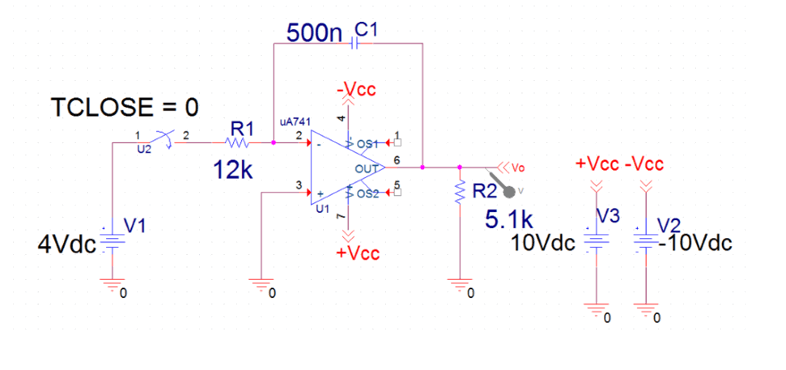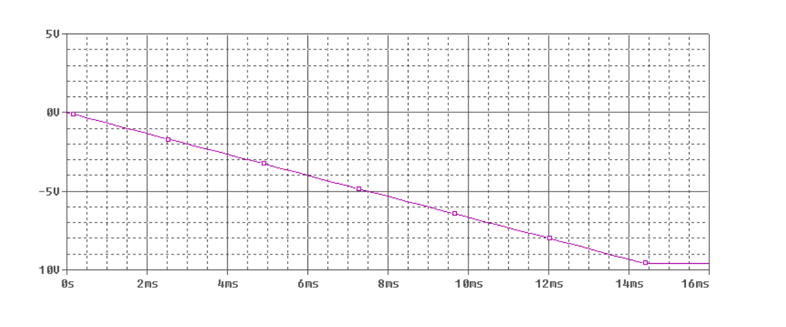The goal is to model an operational amplifiers in PSpice and graph the voltage as a function of time.

# DC Op Amps

Op Amps can add, subtract, multiply or divide input voltage signals. This project analyzes the negative voltage rise of a resistor, capacitor, and op amp circuit. Below is a schematic constructed in PSpice.## Ideal Op Amp Characteristics

At saturation, the magnitude of voltage output equals the magnitude of the power voltage of the Op Amp ($\left|V_o\right|=\left|V_{cc}\right|$ ). Ideally the input internal resistance of the op amp is infinite and the output internal resistance is 0. Therefore, the “positive” voltage input is equal to the “negative” voltage input. These are called ideal characteristics. $$V_p=V_n$$ $$R_{in}\rightarrow \infty$$ $$R_{out}\rightarrow 0$$ $$-V_{cc}=\le V_o\le V_{cc}$$ General procedure to solve for the output voltage
1. Solve for $V_p$ or $V_n$ (whichever is not connected to $V_o$).
2. Use Node-Voltage Method for the one connected to $V_o$.
3. Substitute Equation from step (1) and (2) and solve.
Solving for time till amplifier is saturated $$V_p=0=V_n$$ $$\frac{V_n-4}{12\cdot 10^3}+\frac{d}{dt}\left(V_n-V_o\right)\cdot \left(500\cdot 10^{-9}\right)=0$$ $$\frac{-4}{12\cdot 10^3}-\frac{d}{dt}\left(V_o\right)\cdot \left(500\cdot 10^{-9}\right)=0$$ $$\frac{d}{dt}\left(V_o\right)=-\frac{4}{12\cdot \:10^3\left(500\cdot \:\:10^{-9}\right)}=-\frac{2000}{3}$$ $$V_o=-\frac{2000}{3}t$$ $$-10=-\frac{2000}{3}t\:\rightarrow \:t=.015$$ The graph generated from the PSpice simulation confirms these results.